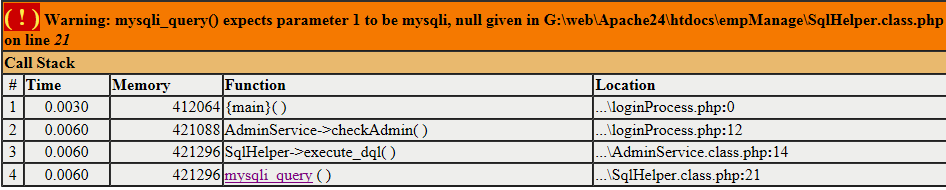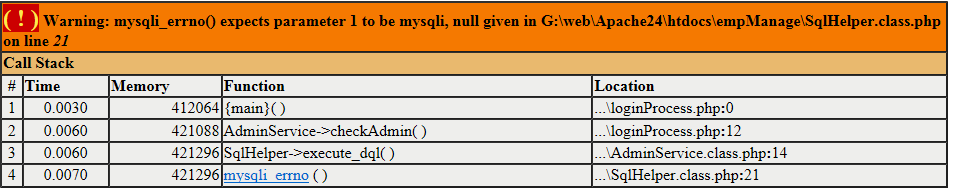# Warning: mysqli_query() expects parameter 1 to be mysqli, null given in XXXXXX问题解决<?php //工具类,完成对数据库操作 class SqlHelper{ public $conn; public$dbname="empmanage"; public $username="root"; public$password="123456"; public $host="localhost"; public function _construct(){$this->conn=mysqli_connect($this->host,$this->username,$this->password,$this->dbname); if (!$this->conn){ die("连接失败".mysqli_errno($this->conn)); } } //执行dql语句 public function execute_dql($sql){$res=mysqli_query($this->conn,$sql) or die(mysqli_errno($this->conn)); return$res; } //执行dml语句 public function execute_dml($sql){$b=mysqli_query($this->conn,$sql); if(!$b){ return 0; }else{ if (mysqli_affected_rows($this->conn)>0){ return 1;//执行成功 }else { return 2;//表示没有行受到影响 } } } //关闭连接 public function close_connect(){ if(!empty($this->conn)){ mysqli_close($this->conn); } } } ?>

<?php require_once 'SqlHelper.class.php'; //该类完成admin表操作 class AdminService{ //提供验证用户合法的方法 public function checkAdmin($id,$password){ $sql = "select password,name from admin where id=$id"； $sqlHelper=new SqlHelper();$sqlHelper->_construct(); $res=$sqlHelper->execute_dql($sql); if($row=mysqli_fetch_assoc($res)){ if(md5($password)==$row['password']){ return$row['name']; } } mysqli_free_result($res);$sqlHelper->close_connect(); return ""; } } ?>

02-242万+
07-314073

03-182318
11-292549
03-1119
04-103963
12-21230
08-16260
02-1125
03-27104
06-123031
02-03134
04-122万+

### “相关推荐”对你有帮助么？

•非常没帮助
•没帮助
•一般
•有帮助
•非常有帮助¥2 ¥4 ¥6 ¥10 ¥20余额支付 (余额：-- )扫码支付获取中扫码支付点击重新获取扫码支付1.余额是钱包充值的虚拟货币，按照1:1的比例进行支付金额的抵扣。
2.余额无法直接购买下载，可以购买VIP、C币套餐、付费专栏及课程。余额充值